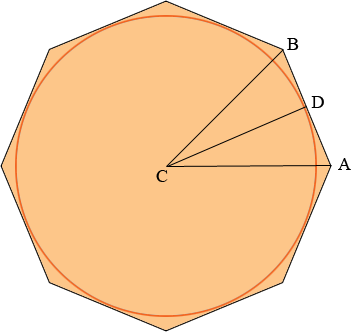SEARCH HOMEMath Central Quandaries & QueriesQuestion from Eric, a parent: I have a circle inscribed in a regular octagon. How do I determine the length of one side of the octagon if I know the radius of the circle (2.75 inches) ?Hi Eric,What is the length of the line segment CA?
What is the measure of the angle BCA?
What is the measure of the angle DCA?
What is the measure of the angle ADC?
What trig function relates the measure of the angle DCA, The side length CA and the side length AD?

PennyMath Central is supported by the University of Regina and The Pacific Institute for the Mathematical Sciences.